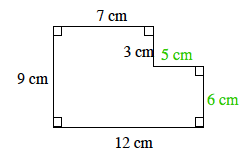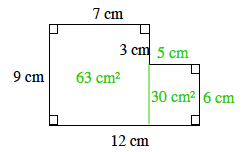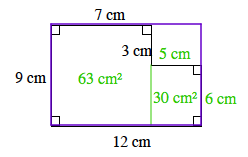### Home > MC1 > Chapter 4 > Lesson 4.2.1 > Problem4-56

4-56.

. Find the area of the shape below in at least two ways. Homework Help ✎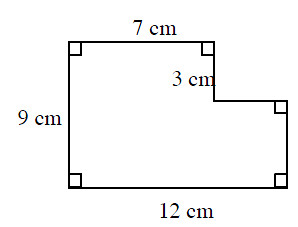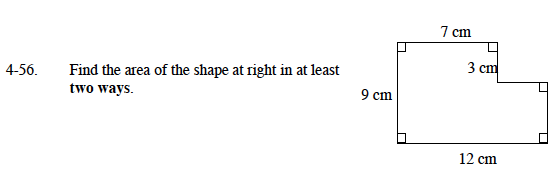Label each remaining side using centimeters.

Our first method of finding the area is to divide the shapeinto smaller rectangles and label their areas.

(9)(7) + (5)(6) = 63 + 30 = 93 square centimeters

Another method for finding the area is to subtract the area of the rectangle in the upper-right corner from the area of the purple rectangle. Show that this method gives the same answer as Method 1.